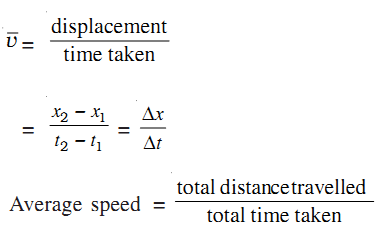When an object travels a certain distance with different velocities, its motion is specified by its average velocity. The average velocity of an object is defined as the displacement per unit time.

Let x1 and x2 be its positions at instants t1 and t2, respectively. Then mathematically average velocity is expressed asThe average speed of an object is obtained by dividing the total distance traveled by the total time taken.